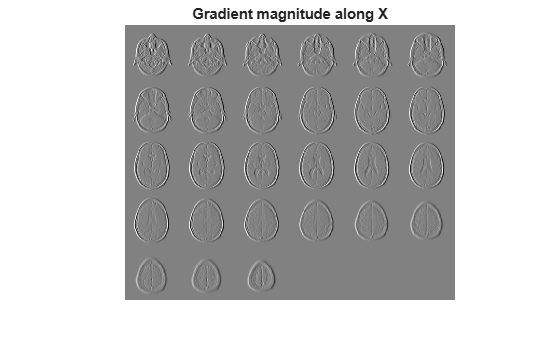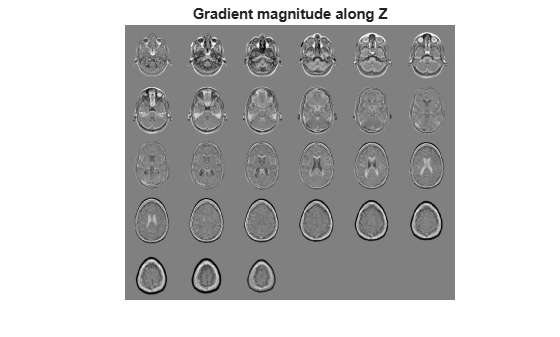Find directional gradients of 3-D image

Description

example

[Gx,Gy,Gz] = imgradientxyz(I) returns the directional gradients Gx, Gy, and Gz of the 3-D grayscale or binary image I.

Examples

collapse all

Read 3-D data and prepare it for processing.

sz = volData.siz;
vol = squeeze(volData.D);

Visualize the directional gradients as a montage.

figure, montage(reshape(Gx,sz(1),sz(2),1,sz(3)),'DisplayRange',[])figure, montage(reshape(Gy,sz(1),sz(2),1,sz(3)),'DisplayRange',[])figure, montage(reshape(Gz,sz(1),sz(2),1,sz(3)),'DisplayRange',[])Input Arguments

collapse all

Input image, specified as a 3-D grayscale image or 3-D binary image.

Data Types: single | double | int8 | int16 | int32 | int64 | uint8 | uint16 | uint32 | uint64 | logical

Gradient operator, specified as one of the following values.

Value

Meaning

'sobel'

Sobel gradient operator. The gradient of a pixel is a weighted sum of pixels in the 3-by-3-by-3 neighborhood. For example, in the depth (z) direction, the weights in the three planes are:

plane z-1plane zplane z+1
[ 1  3  1
3  6  3
1  3  1 ]
[ 0  0  0
0  0  0
0  0  0 ]
[ -1  -3  -1
-3  -6  -3
-1  -3  -1 ]

'prewitt'

Prewitt gradient operator. The gradient of a pixel is a weighted sum of pixels in the 3-by-3-by-3 neighborhood. For example, in the depth (z) direction, the weights in the three planes are:

plane z-1plane zplane z+1
[ 1  1  1
1  1  1
1  1  1 ]
[ 0  0  0
0  0  0
0  0  0 ]
[ -1  -1  -1
-1  -1  -1
-1  -1  -1 ]

'central'

Central difference gradient. The gradient of a pixel is a weighted difference of neighboring pixels. For example, in the depth (z) direction, dI/dz = (I(z+1) - I(z-1))/2.

'intermediate'

Intermediate difference gradient. The gradient of a pixel is the difference between an adjacent pixel and the current pixel. For example, in the depth (z) direction, dI/dz = I(z+1) - I(z).

When applying the gradient operator at the boundaries of the image, imgradientxyz assumes values outside the bounds of the image are equal to the nearest image border value. This behavior is similar to the 'replicate' boundary option in imfilter.

Data Types: char | string

Output Arguments

collapse all

Horizontal gradient, returned as a numeric matrix of the same size as image I. The horizontal (x) axis points in the direction of increasing column subscripts. Gx is of class double, unless the input image I is of class single, in which case Gx is of class single.

Data Types: single | double

Vertical gradient, returned as a numeric matrix of the same size as image I. The vertical (y) axis points in the direction of increasing row subscripts. Gy is of class double, unless the input image I is of class single, in which case Gy is of class single.

Data Types: single | double

Depth gradient, returned as a 3-D numeric array of the same size as image I. The depth (z) axis points in the direction of increasing plane subscripts. Gz is of class double, unless the input image I is of class single, in which case Gz is of class single.

Algorithms

imgradientxyz does not normalize the gradient output. If the range of the gradient output image has to match the range of the input image, consider normalizing the gradient image, depending on the method argument used. For example, with a Sobel kernel, the normalization factor is 1/44, for Prewitt, the normalization factor is 1/18.